The Second Book of the Cross-Staff

Of the use of the former Lines of Proposition more particularly exemplified in several kinds.

The former Book containing the general use of each Line of Proportion, may be sufficient for all those which know the Rule of Three, and the doctrine of Triangles.

But for others, I suppose it would be more difficult to find either the Declination of the Sun, or his Amplitude, or the like, by that which hath been said in the use of the Line of Sines, unless they may have the particular proportions, by which such propositions are to be wrought.

And therefore for their sakes I have adjoined this second Book, containing several proportions for propositions of ordinary use, and set them down in such order, that the Reader considering which is the first of the three Numbers given, may easily apply them to the Sector, and also resolve them by Arithmetick, beginning with those which require help only of the Line of Numbers.

Chap. I.The use of the Line of Numbers in broad measure, such as Board, Glass, and the like.

The ordinary measure for breadth and length are feet and inches, each foot divided into 12 inches, and every inch into halves and quarters, which being parts of several denominations doth breed much trouble both in Arithmetick and the use of instruments.

For the avoiding whereof, where I may prevail I give this counsel, that such as are delighted in measure would use several Lines, first a Line of inche measure, wherein every inch may be divided into 10 or 100 parts; and secondly, a Line of foot measure, wherein every foot may be divided into 100 or 1000 parts, both which Lines may be set on the same side of a two foot Ruler, after this or the like manner.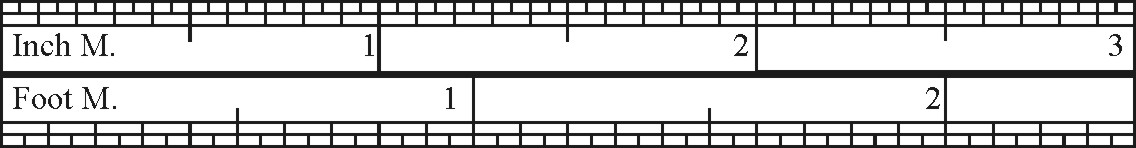Then if they be to give the content of any Superficies or Solid in inches, they may measure the sides of it by the Line of inches and parts of inches; but if they be to give the content in feet, it would be more easie for them to measure those sides by the foot Line and his parts.

For example, let the length of a Plain be 30 inches, and the breadth 21 inches and 6 /24 of an inch; this length multiplied into the breadth, would give the content to be 648 inches: but if I were to find the content of the same Plane in feet, I would measure the sides of it by the foot Line and his parts; so the length would prove to be 2 feet 50/100, and the breadth one foot 30/100, and the length multiplied by the breadth, cutting off the four last figures, for the four figures of the parts, would give the content to be 4.5000, which is 4 foot and 5000 parts of a foot, divided 1000:2000 parts.

 21.6 2.5 30.0 1.8 948.00 10000 250 4.5

The like reason holdeth for Yards and Ells, and all other measures divided into 10.100 or 1000 parts.

This being presupposed, the same work will be more easie both by Arthmetick and the line of Numbers, as may appear by these Propositions.

SECT. I.Of the Mensuration of Oblong Surfaces, and Triangles.

1. Having the breadth and length of an Oblong Superficies given in inch-measure, to find the content in inches.

• As one inch unto the breadth in inches,
• So the length in inches unto the Content in inches.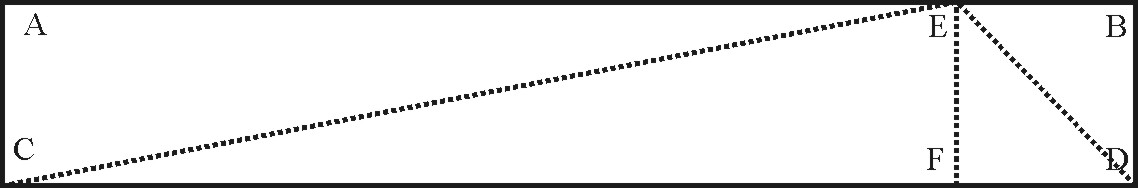Suppose in the Plane AD, the breadth AC to be 30 inches, and the length AB to be 183 inches; extend the Compasses from 1 unto 30, the same extent will reach from 183 unto 5490; or extend them from 1 unto 183 the same extent will reach from 183 unto 5490. So both ways the content required is found to be 5490 inches.

• As 1 unto 30: so are 183 unto 5490.

2. Having the breadth and length of any Oblong Superficies given in inches, to find the content in feet.

• As 144 inches unto the breadth in inches:
• So the length in inches unto the content in feet.

And thus in the former Plane AD, working as before, the content will be found to be 38.125, which is 38 foot and ½ of a foot.

• As 144 unto 30, so are 183 unto 138.125.

3. Having the length and breadth of nay Oblong Superficies given in foot measure, to find the content in feet.

• As 1 foot unto the breadth in foot measure:
• So the length in feet unto the content in feet.

And thus in the former Plane AD, the breadth will be two feet 50 parts, and the length 15 feet 25 parts; then working as before, the content will be found to be 38.125.

• As 1 unto 2.50: so are 15.25 unto 38.125.

4. Having the breadth of any Oblong Superficies given in inches, and the length in foot measure, to find the content in feet.

• As 12 inches to the breadth in inches:
• So the length in feet to the content in feet.

So also in the former Plane, the content will be found to be 38.125.

• As 12 unto 30: so are 15.25 unto 38.125.

5. Having the breadth of an Oblong Superficies given in inches, to find the length of a foot superficial in inch measure.

• As the breadth in inches, unto 144 inches:
• So 1 foot unto the length in inch measure.

So the breadth being 30 inches, the length of a foot will be found to be 4 inches 80 parts, the length of two feet 9 inches 60 parts.

• As 30 unto 144: so are 1 unto 480.

6. Having the breadth of an Oblong Superficies given in feet, to find the length of a foot superfacial in foot measure.

• As the breadth in foot measure to 1 foot:
• So the number of feet to the length in foot measure.

So the breadth being 2 feet 50 parts, the length of a foot will be found to be 40 parts, the length of 2 feet 80 parts, and the length of 3 feet 1 foot 20 parts, &c.

• As 250 unto 1: so are 1 unto 0,40.

7. A four sided Superficies having any of the two sides Parallels, to find the Area.

Add the two Parallel sides together, and take the half, then say,

• As 1 is to the half sum of the two Parallel sides:
• So is the breadth (or length) to the Area.

So in the four sided figure A having one of the parallel sides in length 23.25 foot, and the other, 19.75 foot, the sum of them is 43.00 foot, (the half whereof is 21.50 foot) and the breadth 14.5, the Area or content of this Superficies will be found to be 311.75 foot.

Extend the Compasses from 1 to 21.50 (the mean length) the same extent will reach from 14.5 (the breadth) to 311.15 the Area or content.

8. To find the Area or content of a Triangle, the longest side and the Perpendicular being given.

• As 1 is to the half length of the Base:
• So is the length of the Perpendicular to the Content or Area.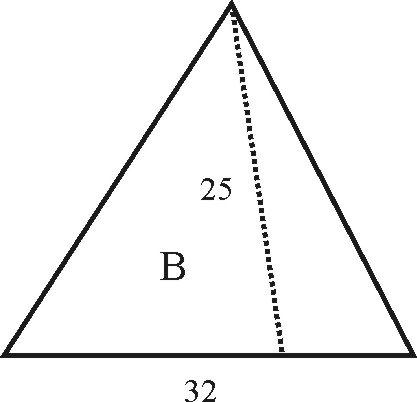So the Triangle B, having the Base 32 foot, and the Perpendicular 25 foot, the Area will be found to be 400 foot.

Extend the Compasses from 1 to 16, (half the Base) the same extent will reach from 25 (the Perpendicular) to 400 the Area.

Or, extend the Compasses from 1 to 12,5. (half the length of the perpendicular) the same extent will reach from 32 (the whole Base) to 400 as before.

Or, extend the Compasses from 1 to 32, the same extent will reach from 25 to 800, the double of the Area.

9. The side of an Equilateral Triangle being given, to find the Area.

• As 1000, is to 433.01,
• So is the Square of the side of the Triangle, to the Area.

So the side of an Equilateral Triangle being 17,5 foot, the Area will be found to be 132.61 foot.

Extend the Compasses from 1000 to 433.01, the same Extent will reach from 306.25 (the Square of the side of the Triangle) to 132.61, the Area.

10. To find the Area of a four sided figure, whose sides are neither equal nor parallel one to the other, which figures are called Trapezias.

All four sided figures whose sides are neither equal nor parallel, must be reduced into two Triangles, by drawing a Diagonal Line from any one Angle to its opposite, upon which Diagonal two perpendiculars must be let fall; then,

• As 1, is to half the length of the Diagonal:
• So is the length of both the perpendiculars, to the Area, or Content.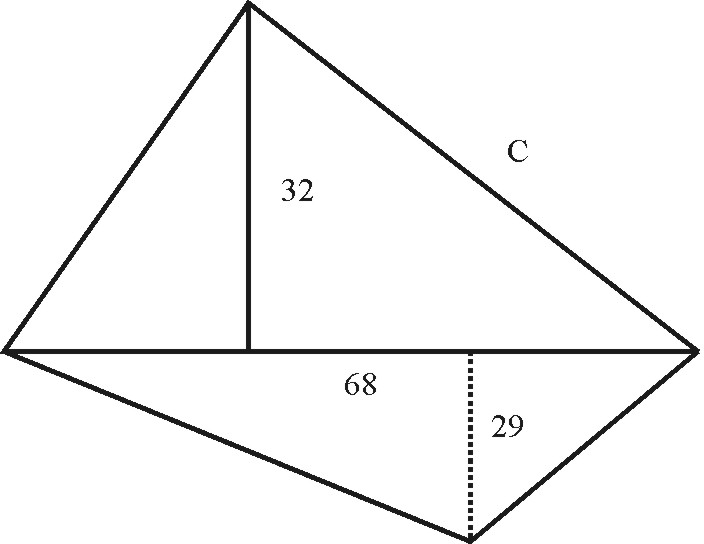So in the Trapezia C, the Diagonal is 68, (the half of it is 34) and the two perpendiculars are 32 and 19, their Sum is 51. Then, extend the Compasses from 1 to 34. (half the Diagonal) the same extent will reach from 51 (the sum of the Perpendiculars) to 1734 the Area.

Or, extend the Compasses from 1 to 68 (the Diagonal) the same extent will reach from 25.5 (half the sum of the two Perpendiculars) to 1734 as before.

In all right lined figures, of how many sides, or how irregular soever, before they can be measured they must (by drawing of Lines from Angle to Angle) be reduced into Triangles or Trapezias, and so be measured by these two last Precepts.

And here note, That when any irregular figure is thus reduced into Triangles, the number of Triangles will be less by two than the number of the sides of the irregular figure.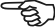11. Having the length and breadth of an Oblong Superficies, to find the side of a Square equal to the Oblong.

Divide the space between the length and the breadth into two equal parts, and the foot of the Compasses will stay at the side of the square.

So the length being 183 inches, and the breadth 30 inches, the side of the square will be found to be 74 inches, and almost 10 parts of 100.

Or the breadth being 2 foot and 50 parts, the length 15 foot and 25 parts, the side of the square will be found to be about 6 feet and 17 parts.

• As 30 unto 70,10: so are 74,10 unto 183,027.
• And as 2,50 unto 6,174: so are 6,174 unto 15,247.

SECT. II.Of the Mensuration of Regular Polygons.

By Regular Polynoms are meant all such figures, whose sides and Angles are above four, and are all equal. As the

 Pentagon Which consisteth of 5 Equal sides and Angles. Hexagon 6 Heptagon 7 Octagon 8 Nonagon 9 Decagon 10

And the Area of any of these Regular Polygons is equal to a Parallelogram, whose length shall be equal to half the Perimeter, and whose breadth equal to a Perpendicular drawn from the Center of the figure to the middle of any of the sides of the Polygon.

1. The side and Perpendicular of a Pentagon being given, to find the Area.

• As 1 is to the Perpendicular 8,258;
• So is half the Perimeter 30 in to the Area 247.74 inches.So in this Pentagon, where the side CD (and so all the rest) contains 12 inches, and the Perpendicular HZ, 8,258 inches, the Area will be found to be 247.74.

Extend the Compasses from 1 to 8.258, (the Perpendicular) the same extent will reach from 30 (which is half the length of all the 5) to 247.74 the Area.

2. The Side and Perpendicular of an Octagon (or figure of 8 sides) being given, to find the Area.

• As 1 to the Perpendicular 14.48.
• So is the Semi-Perimeter 48. to 695.04 the Area.

So a regular Polygon of 8 sides, each side containing 12 inches and the perpendicular 14.48 inches, the Area thereof will be found to be 695.05.

Extend the Compasses from 1to 14.48 the Perpendicular, the same extent will reach from 48 (half the Perimeter) to 695.04, the Area.

And in this nature, may any Regular Polygon, of what number of sides soever, be measured.

SECT. III.Of the Mensuration of Circles.

The Proportion of the Diameter of a Circle to its Circumference as 7 is to 22, but Ludolph Van Ceulen comes somewhat nearer, allowing the Diameter to the Circumference to be (near) as 113 to 355, which proportions I shall use in the following Problems.

1. The Diameter of a Circle being given, to find the Circumference.

• As 113 is to 355:
• So the Diameter to the Circumference.

So the Diameter of a Circle being 15 inches, the Circumference will be found to be 47.12.

Extend the Compasses from 113 to 355, the same extent will reach from 15 the Diameter, to 47,12 inches the Circumference.

2. The Circumference of a Circle being given, to find the Diameter.

• As 355 is to 113:
• So the Circumference to the Diameter.

So the Circumference being 47.12, the Diameter will be found to be 15 inches.

Extend the Compasses from 355 to 113, the same extent will reach backwards from 47.12 to 15.

3. The Diameter of a Circle being given, to find the Area.

• As 28 is to 22:
• So the square of the Diameter 225 to the Area 176.61.

So the Diameter being 15 inches, the Area will be found to be 176.61.

Extend the Compasses from 28 backwards to 22, the same extent applied (the same way) will reach from 225 (the Square of the Diameter) to 176.61, the Area.

4. The Area of a Circle being given, to find the Diameter.

• As 22 is to 28:
• So is the Area 176.61, to the Square of the Diameter 225.

So the Area of a Circle being 176.61 inches, the Diameter will be found to be 15 inches.

Extend the Compasses from 22 to 28, the same extent will reach from 176.61 to 2247.08 the Square of the Diameter, the middle way upon the Line between 2247.08 and 1, is 15 the Diameter.

5. The Circumference of a Circle being given, to find the Area.

• As 88 is to 7:
• So is the Square of the Circumference 2220.29 to the Area 176.61.

So the Circumference of a Circle being 47.12, the Area will be found to be 176.61 inches.

Extend the Compasses from 88 to 7, the same extent will reach from 2220.29 (the square of the Circumference) to 176.61 the Area.

6. The Area of a Circle being given, to find the Circumference.

• As 7 is to 88:
• So is the Area 176.61, to the Square of the Circumference 2220.29.

So the Area of the Circle being 176.61, the Circumference will be found to be 47.12.

Extend the Compasses from 7 to 88, the same extend will reach from 176.61, the Area to 2220.29, the Square of the Circumference, the half distance between 1 and 2220.29, is 47.12 the Circumference.

7. Having the Diameter of a Circle, t find the side of a Square equal to that Circle.

• As 10000 to the Diameter:
• So 8862 unto the side of the Square.

So the Diameter of a Circle being 15 inches, the side of the square will be found to be about 13 inches and 29 parts.

• As 10000 unto 8862: so are 15 unto 29.

8. Having the Circumference of a Circle, to find the side of a Square equal to that Circle.

• As 10000 to the Circumference:
• So 2821 to the side of the Square.

So the Circumference of a Circle being 47 inches 13 parts, the side of the Square will be about 13 inches 29 parts.

• As 10000 unto 2821: so are 47,13 unto 13,29.

SECT. IV.Of the Mensuration of Land by Perch and Acres.

1. Having the breadth and length of an Oblong Superficies, given in Perches, to find the content in Perches.

• As 1 Perch, to the breadth in Perches:
• So the length in Perches, to the content in Perches.

So in the former Plane AD, if the breadth AC be 30 Perches, and the length AB 183 Perches, the content will be found to be 5490 Perches.

2. Having the length and breadth of an Oblong Superficies given in Perches, to find the content in Acres.

• As 160, to the breadth in Perches:
• So the length in Perches, to the content in Acres.

So in the former Plane AD, the content will be found to be 34 Acres, and 31 Centesms, or parts of 100.

• As 160, unto 30: So are 183, unto 34,31.

To augment a Superficies in a proportion.

To diminish a Superficies in a proportion.

 * Centesms of a Chain Long. Inch. Cent.* Foot. Pace. Perch. Chain. Acre. Mile. Inch 1 7,92 12 60 198 792 7920 63360 Cent. 62,7264 1 1,515 7,575 25 100 1000 8000 Foot. 144 2,295 1 5 26,5 66 660 5280 Pace. 3600 57,485 25 1 3,3 13,2 132 1056 Perch. 39204 625 272,25 10,89 1 4 40 320 Chain. 627164 10000 4356 17484 16 1 10 80 Acre. 6272640 100000 43560 1742,4 160 0 1 8 Mile. 4014489600 64000000 27878400 1115136 10400 6400 640 1 Squares

3. Having the length and breadth of an Oblong Superficies given in Chains, to find the content in Acres.

It being troublesome to divide the content in Perches by 160, we may measure the length and breadth by chains, each chain being 4 Perches in length, and divide into 100 links, then will the work be more easie in Arithmetick. For,

• As 10 to the breadth in Chains:
• So the length in Chains, to the content in Acres.

And thus in the former Plane AD, the breadth AC will be 7 Chains 50 Links, and the length AB 45 Chains 75 Links; then working as before, the content will be found as before, 34 Acres 31 parts.

4. Having the Perpendicular and Base of a Triangle given in Perches, to find the content in Acres.

If the Perpendicular go for the breadth, and the Base for the length, the Triangle will be the half of the Oblong, as the Triangle CED is the half of the Oblong AD, whose content was found in the former Proposition. Or without halfing:

• As 320 to the Perpendicular:
• So the Base, to the content in Acres.

So in the Triangle CED, the Perpendicular being 30, and the Base 183, the content will be found 17 Acres and 15 parts.

5. Having the Perpendicular and Base of a Triangle given in Chains, to find the content in Acres.

• As 20 to the Perpendicular:
• So the Base, to the content in Acres.

And so in the Triangle CED, the Perpendicular EF being 7,50, and the Base CD 45,75, the content will be found, as before, to be about 17 Acres 15 parts.

6. Having the content of a Superficies after one kind of Perch, to find the content of the same Superficies, according to another kind of Perch.

• As the length of the second Perch, to the length of the first Perch:
• So the content in Acres to a fourth number; and that fourth to the content in Acres required.

Suppose the Plane AD measured with a chain of 66 feet, or with a Perch of 16 feet and a half, containeth 34 Acres 31 parts; and it were demanded how many Acres it would contain, if it were measured with a chain of 18 foot to the perch: these kind of Propositions are wrought by the backward Rule of three, after a duplicate proportion. Wherefore I extend the Compasses from 16,5 unto 18,0, and the same extent doth reach backward, first from 34,31 to 31,45, and then from 31,45 to 28,84, which shews the content to be 28 Acres 84 parts.

7. Having the plot of a Plane with the content in Acres, to find the Scale by which it was plotted.

Suppose the Plane AD, containeth 34 Acres 31 Centesms; if I should measure it with a Scale of 10 in the inch, the length AB would be 38 Chains, and about 12 Centesms, and the breadth AC, 6 Chains and 25 Centesms; and the content would be found by the third Proposition of this Chapter, to be about 22 Acres 82 parts, whereas it should be 34 Acres 31 parts.

Wherefore I divide the distance between 23,82 and 34,31, upon the Line of Numbers, into two equal parts; then setting one foot of the Compasses upon 10, my supposed Scale, I find the other to extend to 12, which is the Scale required.

8. Having the length of the Furlong, to find the breadth of the Acre.

• As the length in perches, to 160:
• So the Acres to the breadth in perches.

So the length of the Furlong being 40 perches, the breadth for an Acre will hold found to be 4 Perches. If the length be 50, the breadth for one Acre must be 3,20, the breadth for two Acres 6,40.

Or if the length be measured by chains.

• As the length in chains unto 10:
• So 1 Acre to his breadth in chain measure.

So the length of the Furlong being 12 Chains 50 Links the breadth for one Acre will be found to be 80 Links, the breadth for two Acres 1 Chain 60 Links.

• As 12,50, unto 10: so 1 unto 0,80.

Or if the length be measured by feet measure:

• As the length in feet, unto 43560:
• So 1 Acre, to his breadth in foot measure.

So the length of the Furlong being 792 feet, the breadth for one Acre will be found to be 55 feet, the breadth for two Acres 110 feet.

Zurück zum Inhaltsverzeichnis Nächstes Kapitel© Rainer Stumpe URL: http://www.rainerstumpe.de